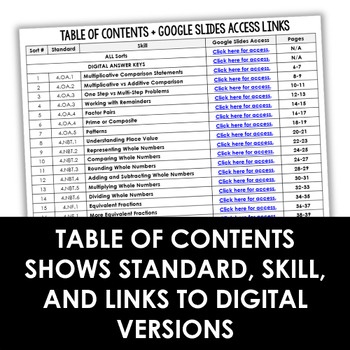Total:
\$0.00# 4th Grade Math Sorts - Digital Math Sorts Included for Digital Math Centers4th
Subjects
Standards
Resource Type
Formats Included
• PDF
•Google Apps™
Pages
36 Math Sorts + Digital VersionsThe Teacher-Author indicated this resource includes assets from Google Workspace (e.g. docs, slides, etc.).

#### Also included in

1. Do you want your math stations and guided math centers done for the year? This 4th grade guided math bundle is the only resource you will need to have your math centers done for the year!Need 3rd or 5th Grade Math Centers?Click here to see the 3rd Grade Guided Math Mega Bundle.Click here to see the
\$95.00
\$168.50
Save \$73.50

### Description

Math sorts are an excellent way to push your students' learning and thinking as they explore new math topics. This print and digital math resource includes 4th grade math sorts aligned to the common core standards (but can be used with other standards as well).

Need 3rd or 5th Grade Math Sorts?

• This resource includes 36 math sorts, one for each standard/substandard for 4th grade common core.

• The math sorts are included in printable format and a digital format for use with Google Slides.

• Answer keys for the math sorts are included in the PDF file.

Each printable math sort is 2 pages: 1 page for the pockets and directions and one page for the sorting cards.

Using the Printable Math Sorts

These sorts work perfectly in an interactive notebook, but would also work in a center. You could even glue the pockets to a file folder and create an instant sorting game for fun review. I have included a few generic recording sheets if you wish to go with this option.

Printing Tip: Print the sorts with four pockets at 70% and the sorts with three pockets at 80% to fit all the pockets on one page in a spiral notebook.

The digital math sorts are included for use with Google Slides.

The math sorts are all separated by skill. The links to access the digital math sorts can be found on pages 3 and 4.

A digital answer key is also included for easy grading of the math sorts or to have students self-check.

4th Grade Math Skills (One Sort Per Skill):

• Multiplicative Comparison Statements
• One Step vs Multi-Step Problems
• Working with Remainders
• Factor Pairs
• Prime or Composite
• Patterns
• Understanding Place Value
• Representing Whole Numbers
• Comparing Whole Numbers
• Rounding Whole Numbers
• Adding and Subtracting Whole Numbers
• Multiplying Whole Numbers
• Dividing Whole Numbers
• Equivalent Fractions
• More Equivalent Fractions
• Comparing Fractions
• Decomposing Fractions
• Adding and Subtracting Mixed Numbers
• Fraction Word Problems
• Multiplying with Fractions
• Working with Fractions with Denominators of 10 and 100
• Fractions and Decimals
• Comparing Decimals
• Comparing Measurement Conversions
• Measurement Word Problems
• Area and Perimeter
• Line Plots (Printable Only)
• Rays and Angles
• Types of Angles
• Types of Triangles
• Types of Lines
• Lines in 2-D Shapes
• Symmetry

Using the 4th Grade Math Sorts

The math sorts could be used as part of your interactive math lesson, for RTI or reteaching, in math centers, tutoring, or even homework.

The students could create a notebook of sorts that they continually practiced and sorted for homework throughout the year.

The digital math sorts can be used as a digital math center, digital homework option, or for distance learning.

Make sure you grab the FREE Launching Math Centers Starter Pack with review centers to help you launch your centers. You can grab it by clicking here.

Total Pages
36 Math Sorts + Digital Versions
Included
Teaching Duration
1 Year
Report this Resource to TpT
Reported resources will be reviewed by our team. Report this resource to let us know if this resource violates TpT’s content guidelines.

### Standards

to see state-specific standards (only available in the US).
Interpret a multiplication equation as a comparison, e.g., interpret 35 = 5 × 7 as a statement that 35 is 5 times as many as 7 and 7 times as many as 5. Represent verbal statements of multiplicative comparisons as multiplication equations.
Multiply or divide to solve word problems involving multiplicative comparison, e.g., by using drawings and equations with a symbol for the unknown number to represent the problem, distinguishing multiplicative comparison from additive comparison.
Solve multistep word problems posed with whole numbers and having whole-number answers using the four operations, including problems in which remainders must be interpreted. Represent these problems using equations with a letter standing for the unknown quantity. Assess the reasonableness of answers using mental computation and estimation strategies including rounding.
Find all factor pairs for a whole number in the range 1-100. Recognize that a whole number is a multiple of each of its factors. Determine whether a given whole number in the range 1-100 is a multiple of a given one-digit number. Determine whether a given whole number in the range 1-100 is prime or composite.
Generate a number or shape pattern that follows a given rule. Identify apparent features of the pattern that were not explicit in the rule itself. For example, given the rule “Add 3” and the starting number 1, generate terms in the resulting sequence and observe that the terms appear to alternate between odd and even numbers. Explain informally why the numbers will continue to alternate in this way.